Courses

# Methods of Ray-Tracing Physics Notes | EduRev

## Physics : Methods of Ray-Tracing Physics Notes | EduRev

The document Methods of Ray-Tracing Physics Notes | EduRev is a part of the Physics Course Basic Physics for IIT JAM.
All you need of Physics at this link: Physics

Graphical Ray Tracing:

In 1621 Willebrord Snell, a professor of mathematics at Leiden, discovered a simple graphical procedure for determining the direction of the refracted ray at a surface when the incident ray is given. The mathematical form of the law of refraction, equation (1) above, was announced by the French mathematician René Descartes some 16 years later.

Snell’s construction is as follows: The line AP in Figure 3A represents a ray incident upon a refracting surface at P, the normal at P being PN. If the incident and refracted rays are extended to intersect any line SS parallel to the normal, the lengths PQ and PR along the rays will be proportional to the refractive indices n and n′. Hence, if PQ and the indices are known, PR can be found and the refracted ray drawn in.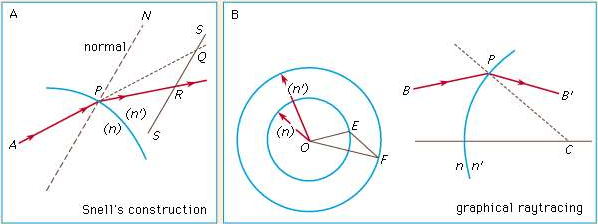Figure 3A: Graphic refraction procedures (see text).

A convenient modification of Snell’s construction can readily be used to trace the path of a ray through a complete lens. In Figure 3B, the incident ray BP strikes a refracting surface at P. The normal to the surface is PC. At any convenient place on the page two concentric circles are drawn about a point O with radii proportional to the refractive indices n and n′, respectively. A line OE is now drawn parallel to the incident ray BP extending as far as the circle representing the refractive index n of the medium containing the incident ray. From E a line is drawn parallel to the normal PC extending to F on the circle representing the refractive index n′. The line OF then represents the direction of the desired refracted ray, which may be drawn in at PB′. This process is repeated successively for all the surfaces in a lens. If a mirror is involved, the reflected ray may be found by drawing the normal line EF across the circle diagram to the incident-index circle on the other side.

Trigonometrical Ray Tracing:

No graphical construction can possibly be adequate to determine the aberration residual of a corrected lens, and for this an accurate trigonometrical computation must be made and carried out to six or seven decimal places, the angles being determined to single seconds of arc or less. There are many procedures for calculating the path of a ray through a system of spherical refracting or reflecting surfaces, the following being typical: The diagram in Figure represents a ray lying in the meridian plane, defined as the plane containing the lens axis and the object point. A ray in this plane is defined by its slope angle, U, and by the length of the perpendicular, Q, drawn from the vertex (A) of the surface on to the ray. By drawing a line parallel to the incident ray through the centre of curvature C, to divide Q into two parts at N, the relation is stated as AN = r sin U, and NM = r sin I. Hence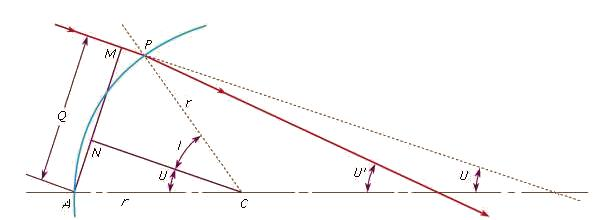Figure 4: Trigonometrical ray tracing (see text).

Q = r(sin U + sin I)      (2)

From this the first ray-tracing equation can be derived,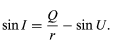(3a)

Applying the law of refraction, equation (2), gives the second equation

Q = r(sin U + sin I)      (2)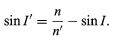(3b)

Because the angle PCA = U + I = U′ + I′, the slope of the refracted ray can be written as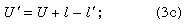and, lastly, by adding primes to equation (2)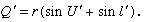in which d is the axial distance from the first to the second refracting surface. After performing this calculation for all the surfaces in succession, the longitudinal distance from the last surface to the intersection point of the emergent ray with the lens axis is found by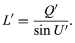Corresponding but much more complicated formulas are available for tracing a skew ray, that is, a ray that does not lie in the meridian plane but travels at an angle to it. After refraction at a surface, a skew ray intersects the meridian plane again at what is called the diapoint. By tracing the paths of a great many (100 or more) meridional and skew rays through a lens, with the help of an electronic computer, and plotting the assemblage of points at which all these rays pierce the focal plane after emerging from the lens, a close approximation to the appearance of a star image can be constructed, and a good idea of the expected performance of a lens can be obtained.

Offer running on EduRev: Apply code STAYHOME200 to get INR 200 off on our premium plan EduRev Infinity!

,

,

,

,

,

,

,

,

,

,

,

,

,

,

,

,

,

,

,

,

,

;